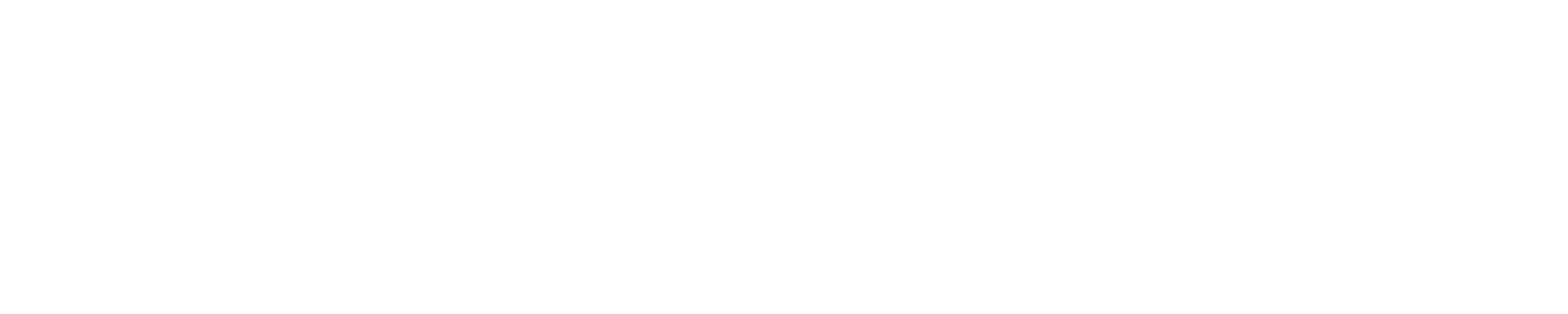I’m trying to modify the ADS component to suit my needs.
2 things I’m trying to accomplish:

• Create an update interval parameter, so the entity only gets updated every X seconds
• Do decimal rounding before writing the value to HA

Here is my modified code, a snippet of init.py ADS hub:

``````        def update(name, value):
_LOGGER.debug("Variable %s changed its value to %d", name, value)

# Modification
needsupdate = False

if self._state_dict[state_key] == None:
needsupdate = True
else:
states = self.hass.states.get('sensor.' + self._name)
update_after = dt_util.utcnow() - timedelta(seconds=update_interval)
if update_after > states.last_updated:
needsupdate = True

if needsupdate == True:
if factor is None:
tempvalue = value
else:
tempvalue = value / factor

if round_perform:
x = Decimal(tempvalue)
if round_decimal == 0:
value = round(x, None)
else:
value = round(x, round_decimal)
else:
value = tempvalue

self._state_dict[state_key] = value
self.schedule_update_ha_state()
``````

2 problems I encounter:

• When HA restarts, the log spits out the following error:
``````2020-05-31 01:35:14 ERROR (Dummy-9) [root] Uncaught exception
Traceback (most recent call last):
File "_ctypes/callbacks.c", line 232, in 'calling callback function'